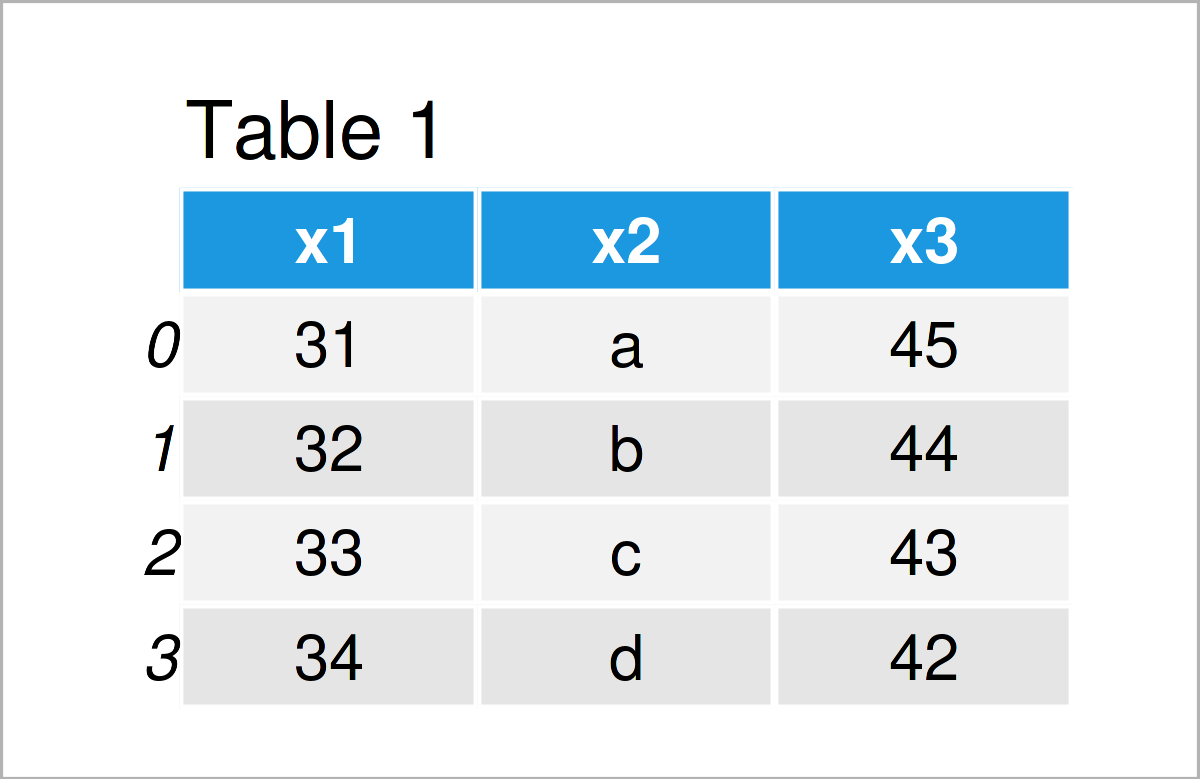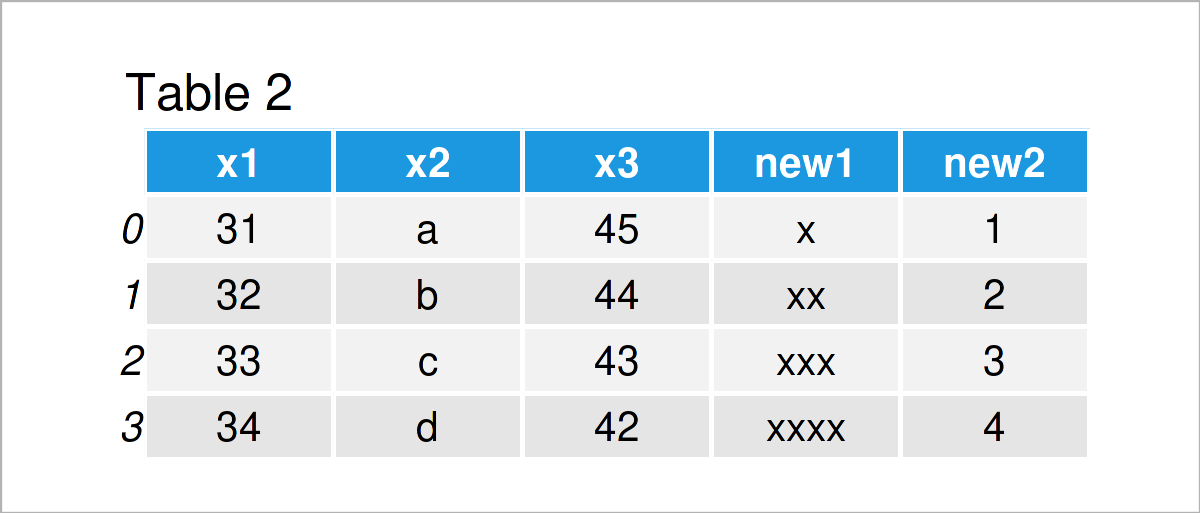# Add Multiple Columns to pandas DataFrame in Python (Example)

In this tutorial, I’ll illustrate how to append multiple new variables to a pandas DataFrame in the Python programming language.

The content is structured as follows:

So let’s take a look at some Python codes in action:

## Example Data & Add-On Libraries

In order to use the functions of the pandas library, we first have to load pandas:

`import pandas as pd                                # Import pandas library`

The following data is used as basement for this Python programming tutorial:

```data = pd.DataFrame({"x1":range(31, 35),           # Create pandas DataFrame
"x2":["a", "b", "c", "d"],
"x3":range(45, 41, - 1)})
print(data)                                        # Print pandas DataFrame```Table 1 shows that our example data consists of four rows and three columns.

Next, we have to create several list objects that we will add as new columns to our pandas DataFrame later on.

```new1 = ["x", "xx", "xxx", "xxxx"]                  # Create first list
print(new1)                                        # Print first list
# ['x', 'xx', 'xxx', 'xxxx']```
```new2 = [1, 2, 3, 4]                                # Create second list
print(new2)                                        # Print second list
# [1, 2, 3, 4]```

Note that our two lists have the same length as the number of rows of our data set.

## Example: Append Multiple Columns to pandas DataFrame

In this example, I’ll demonstrate how to combine multiple new columns with an existing pandas DataFrame in one line of code.

Consider the following python syntax:

```data_new = data.copy()                             # Create copy of DataFrame
data_new["new1"], data_new["new2"] = [new1, new2]  # Add multiple columns
print(data_new)                                    # Print updated pandas DataFrame```By running the previous code, we have created Table 2, i.e. a new pandas DataFrame containing a union of our example data set plus our two list objects.

## Video & Further Resources

In case you need further info on how to merge and join new columns to a pandas DataFrame, you could watch the following video on my YouTube channel. I explain the examples of this article in the video instruction.

Please accept YouTube cookies to play this video. By accepting you will be accessing content from YouTube, a service provided by an external third party.If you accept this notice, your choice will be saved and the page will refresh.

In addition, you may want to read the related tutorials on my website. You can find a selection of tutorials on related topics such as counting and descriptive statistics below: